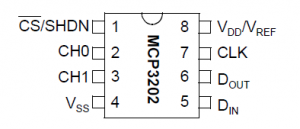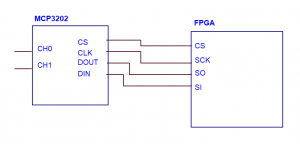# VHDL for Analog to Digital Converter (ADC) MCP3202

The Analog to digital converter (ADC) is functioned to convert an analog signal into a digital signal. In this case, the signal conversion is processed using FPGA. There are some types of ADC in the signal conversion, i.e. Successive approximation ADC, Dual slope ADC, Sigma-delta ADC, Flash ADC, and so on.

In this article presents an ADC of MCP3202 IC. This chip is based on the technique of successive approximation register method (SAR) Conversion. It has only 8 pins which are shown in Fig. 1.Figure 1. Pin Diagram of ADC MCP3202

This ADC will be initiated with the MSB bit of 1. Thus the register monitor comparator output will check if the binary values of SAR greater or less then analog input and adjusted to the binary value according to it. The description of this pins could be shown in below,

1. Shutdown/chip select (SHDN/CS): the SHDN/CS pin is used to initiate the communication between the FPGA and ADC. If this pin is low, the conversation will initiate. If the pin is high, the device will be in standby mode.
2. CH0/CH1: there are two independent channels which are called as CH0/CH1. These channels can be programmed as two independent channels in single mode or a single pseudo-differential input where one is IN+ and another is IN-.
3. Vss: this pin is connected to GND.
4. Serial input (Din): this pin is used as clock in to input channel configuration data.
5. Serial data out (Dout): this pin can be used as shit out the result of the serial conversion. The data will be change on the falling edge of each clock.
6. Serial clock (SCK): the serial clock is used to initiate the conversion and it gives the clock for each bit of conversion.
7. Vdd/Vref: this both pins are common for supply and reference input of the ADC.

Interfacing Between FPGA and ADC MCP3202

There is a clock line of SCK which is connected to the CLK to synchronize the transfer. The clock is always controlled by the FPGA. This communication is a full duplex that the data can be sent and received simultaneously. An SPI transfer is initiated by pulling the CS line in a low state. The CS line sets at HIGH during the idle state. So the FPGA can write to the bus in 8-bit data. The configuration between the FPGA and MCP3202 can be shown in Fig. 2.Figure 2. The configuration of MCP3202 and FPGA